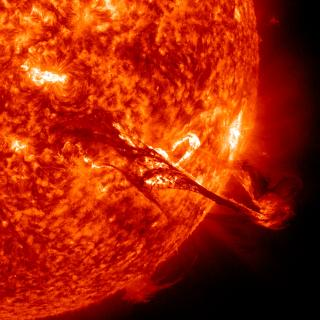MHDSTS: a new explicit numerical scheme for simulations of partially ionised solar plasma

González-Morales, P. A.; Khomenko, E.; Downes, T. P.; de Vicente, A.
Referencia bibliográfica

Astronomy & Astrophysics, Volume 615, id.A67, 14 pp.

Fecha de publicación:
7
2018
Descripción
The interaction of plasma with magnetic field in the partially ionised solar atmosphere is frequently modelled via a single-fluid approximation, which is valid for the case of a strongly coupled collisional media, such as solar photosphere and low chromosphere. Under the single-fluid formalism the main non-ideal effects are described by a series of extra terms in the generalised induction equation and in the energy conservation equation. These effects are: Ohmic diffusion, ambipolar diffusion, the Hall effect, and the Biermann battery effect. From the point of view of the numerical solution of the single-fluid equations, when ambipolar diffusion or Hall effects dominate can introduce severe restrictions on the integration time step and can compromise the stability of the numerical scheme. In this paper we introduce two numerical schemes to overcome those limitations. The first of them is known as super time-stepping (STS) and it is designed to overcome the limitations imposed when the ambipolar diffusion term is dominant. The second scheme is called the Hall diffusion scheme (HDS) and it is used when the Hall term becomes dominant. These two numerical techniques can be used together by applying Strang operator splitting. This paper describes the implementation of the STS and HDS schemes in the single-fluid code MANCHA3D. The validation for each of these schemes is provided by comparing the analytical solution with the numerical one for a suite of numerical tests.